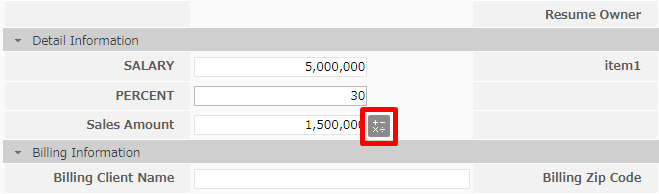# Inter-field calculation for number and currency type

Now, inter-field calculation for number and currency type items is possible.

【Calculation example】

The alias of item 1 is Sales.U_XX1 and the alias of item 2 is Sales.U_XX2.

Please set the calculation formula in item 3.

Addition Total value of items: Sales.U_XX1+Sales.U_XX2                             : Add(Sales.U_XX1,Sales.U_XX2) Add 10 to item 1: Sales.U_XX1+10 Subtract the value of item 2 from the value of item 1: Sales.U_XX1-Sales.U_XX2                                                                             : Sub (Sales.U_XX1,Sales.U_XX2) Subtract item 1 from 100: Sales.U_XX1-100 Multiply the value of item 1 by the value of item 2: Sales.U_XX1*Sales.U_XX2                                                                         : Mul(Sales.UXX1,Sales.U_XX2)  Sales tax calculation: Sales.U_XX1*0.08 Calculation of tax amount: Sales.U_XX1+(Sales.U_XX1*0.08) Divide item 1 by item 2:Sales.U_XX1/Sales.U_XX2                                   :div(Sales.U_XX1,Sales.U_XX2) Calculating tax-free amount:Sales.U_XX1/1.08 Total sum of total items: sum(Sales.U_XX1,Sales.U_XX2,Sales.U_……) Round the value of item 1: round(Sales.U_XX1) * Rounded to the number specified in item setting. Truncate the value of item 1: floor(Sales.U_XX1) * Round down to the number specified in item setting. Round up the value of truncation item 1: ceil(Sales.U_XX1) * Round up to the number specified in item setting. The remainder obtained by dividing item 1 by item 2: mod(Sales.U_XX1,Sales.U_XX2) Square root of item 1 Square root: root(Sales.U_XX1)

【Setting】

Open the setting screen of number type · currency type item and enter calculation formula in [calculation formula].

Only half-size alphanumeric characters and next letters can be used in calculation formulas. + - * /., () _

Items used for calculation are specified by alias.

The alias of the item can be confirmed from the item setting screen on the customize screen.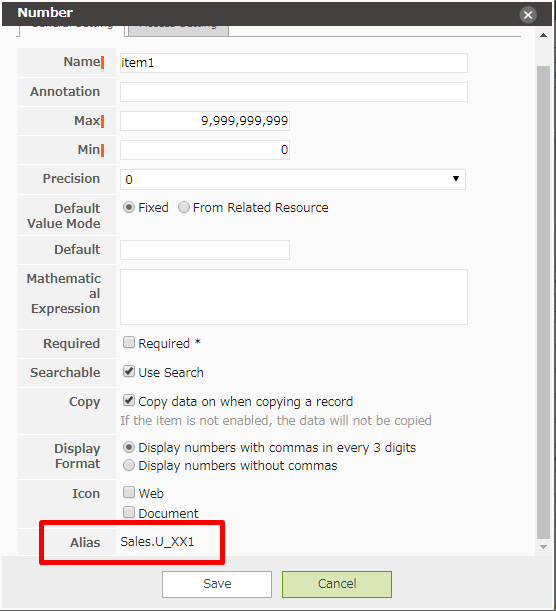For example, if you want to get the sales by multiplying the assumed annual income of a candidate by the compensation rate,You can set as following.

Alias ：Sales.U_SALARY（annual income）

Sales.U_PERCENT（compensation rate）

Calculation formula：Sales.U_SALARY*(Sales.U_PERCENT/100)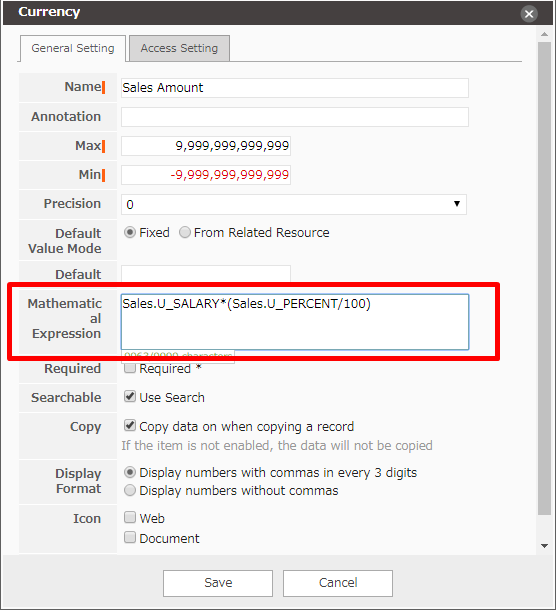When a numerical value is input to the assumed annual income and remuneration rate, the calculation result is automatically input as a value.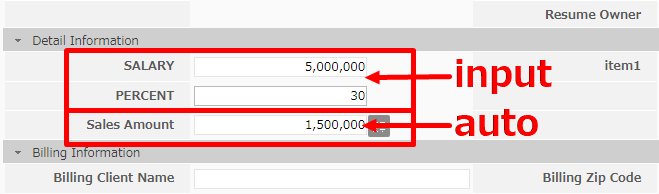When you modify the related items of the calculation formula, "The above fields' values may be affected" will be displayed.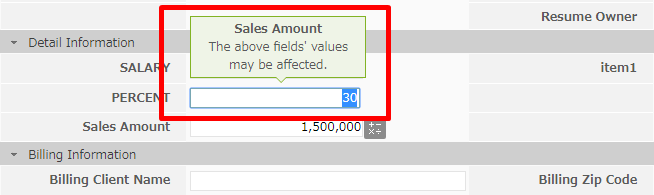When changing the value of the item for which the calculation formula is set, click the "+ - × ÷" button to input the calculation result value again.